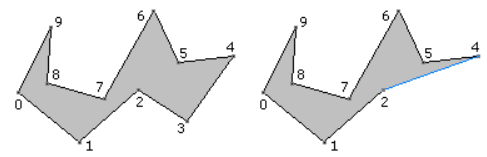# Problem E

## Ears Cutting

A famous way to cut polygon into triangles is ear cutting: each time cut off a triangle along a diagonal, after n-3 cuts only a single triangle remains. In the following picture, the ear {2,3,4} was cut off.Find a way to cut ears of a simple polygon such that the sum of cut lengths is minimal.

## Input

There will be at most 30 test cases. The first line of each case contains the number of vertices, n (4<=n<=100). Each of the following n lines contains the coordinates of a vertex, in clockwise OR counter-clockwise order. Coordinates are integers whose absolute value does not exceed 10000.

## Output

For each test case, print the minimal sum of cut lengths, rounded to 4 decimal digits.

```4
0 0
3 0
1 1
0 3
4
0 0
10 0
10 1
0 1
```

## Output for the Sample Input

```Case 1: 1.4142
Case 2: 10.0499
```

The Ninth Hunan Collegiate Programming Contest (2013)
Problemsetter: Rujia Liu
Special Thanks: Md. Mahbubul Hasan